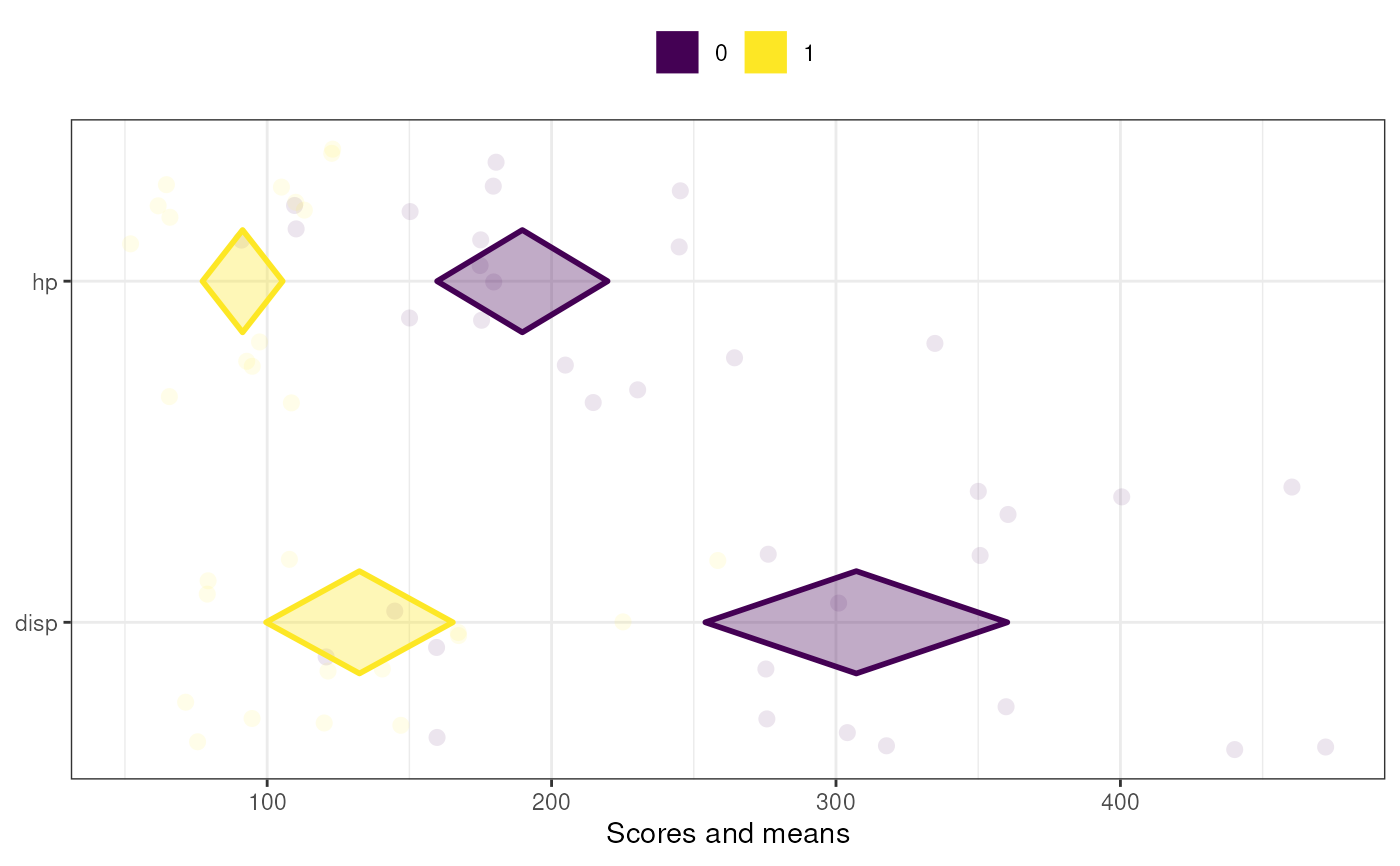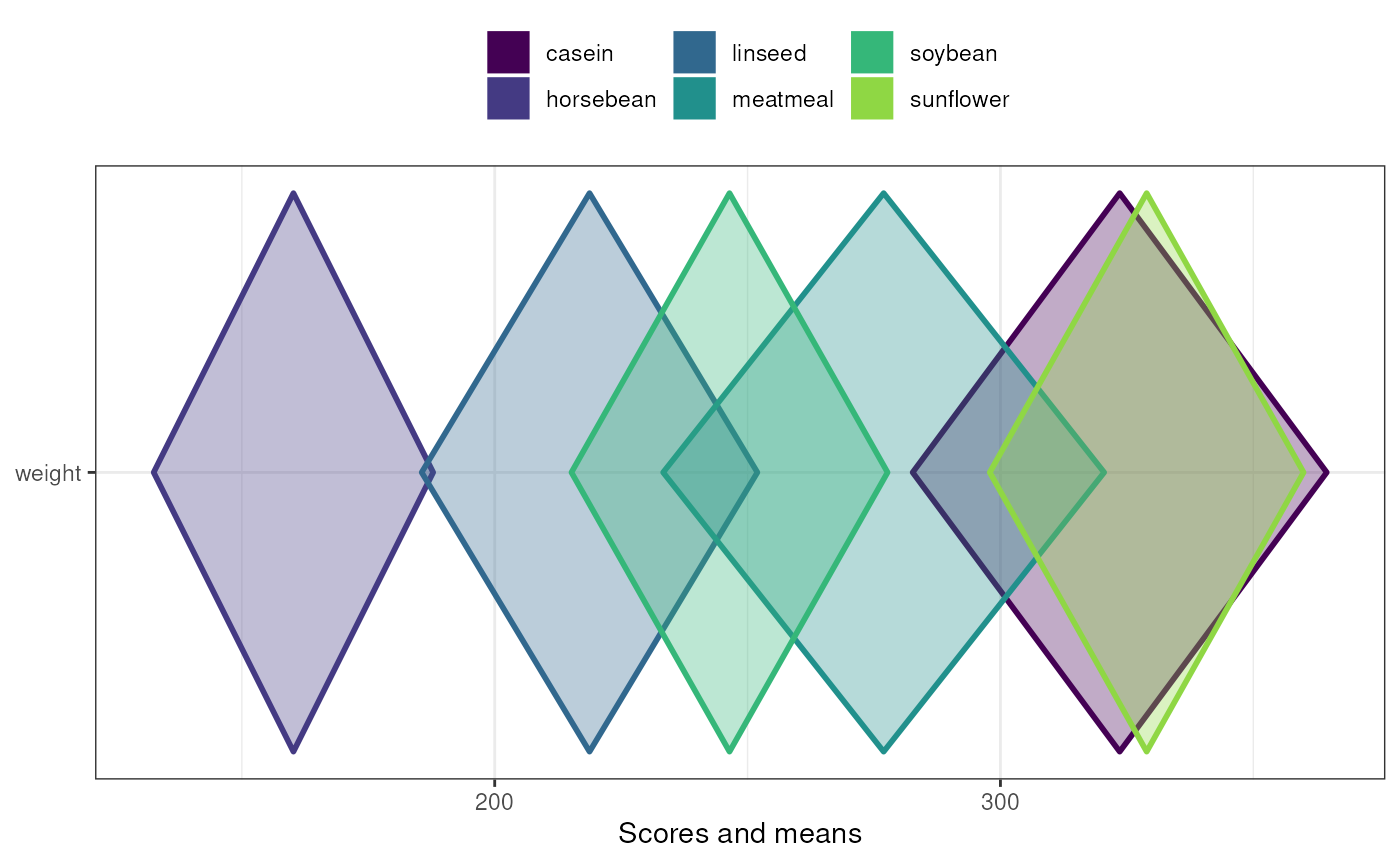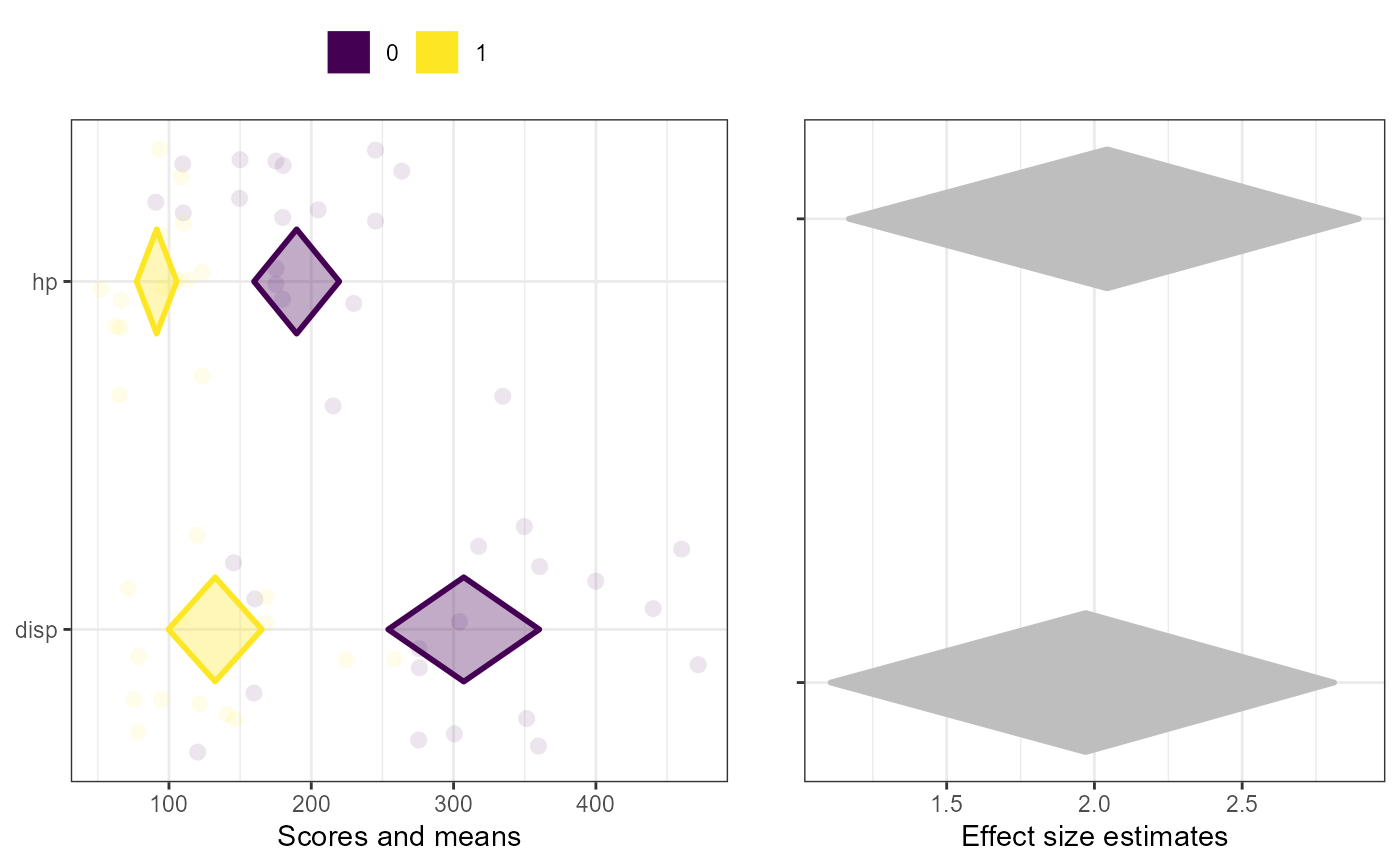These are two diamond plot functions to conveniently make diamond plots to compare subgroups or different samples. They are both based on a univariate diamond plot where colors are used to distinguish the data points and diamonds of each subgroup or sample. The means comparison diamond plot produces only this plot, while the duo comparison diamond plot combines it with a diamond plot visualising the effect sizes of the associations. The latter currently only works for two subgroups or samples, while the simple meansComparisonDiamondPlot also works when comparing more than two sets of datapoints. These functions are explained more in detail in Peters (2017).

duoComparisonDiamondPlot(
dat,
items = NULL,
compareBy = NULL,
labels = NULL,
compareByLabels = NULL,
decreasing = NULL,
conf.level = c(0.95, 0.95),
showData = TRUE,
dataAlpha = 0.1,
dataSize = 3,
comparisonColors = viridisPalette(length(unique(dat[, compareBy]))),
associationsColor = "grey",
alpha = 0.33,
jitterWidth = 0.5,
jitterHeight = 0.4,
xlab = c("Scores and means", "Effect size estimates"),
ylab = c(NULL, NULL),
plotTitle = NULL,
theme = ggplot2::theme_bw(),
showLegend = TRUE,
legend.position = "top",
lineSize = 1,
drawPlot = TRUE,
xbreaks = "auto",
outputFile = NULL,
outputWidth = 10,
outputHeight = 10,
ggsaveParams = ufs::opts$get("ggsaveParams"), ... ) meansComparisonDiamondPlot( dat, items = NULL, compareBy = NULL, labels = NULL, compareByLabels = NULL, decreasing = NULL, sortBy = NULL, conf.level = 0.95, showData = TRUE, dataAlpha = 0.1, dataSize = 3, comparisonColors = viridisPalette(length(unique(dat[, compareBy]))), alpha = 0.33, jitterWidth = 0.5, jitterHeight = 0.4, xlab = "Scores and means", ylab = NULL, plotTitle = NULL, theme = ggplot2::theme_bw(), showLegend = TRUE, legend.position = "top", lineSize = 1, xbreaks = "auto", outputFile = NULL, outputWidth = 10, outputHeight = 10, ggsaveParams = ufs::opts$get("ggsaveParams"),
...
)

## Arguments

dat

The dataframe containing the relevant variables.

items

The variables to plot (on the y axis).

compareBy

The variable by which to compare (i.e. the variable indicating to which subgroup or sample a row in the dataframe belongs).

labels

The labels to use on the y axis; these values will replace the variable names in the dataframe (specified in items).

compareByLabels

The labels to use to replace the value labels of the compareBy variable.

decreasing

Whether to sort the variables by their mean values (NULL to not sort, TRUE to sort in descending order (i.e. items with lower means are plotted more to the bottom), and FALSE to sort in ascending order (i.e. items with lower means are plotted more to the top).

conf.level

The confidence level of the confidence intervals specified by the diamonds for the means (for meansComparisonDiamondPlot) and for both the means and effect sizes (for duoComparisonDiamondPlot).

showData

Whether to plot the data points.

dataAlpha

The transparency (alpha channel) value for the data points: a value between 0 and 1, where 0 denotes complete transparency and 1 denotes complete opacity.

dataSize

The size of the data points.

comparisonColors

The colors to use for the different subgroups or samples. This should be a vector of valid colors with at least as many elements as sets of data points that should be plotted.

associationsColor

For duoComparisonDiamondPlot, the color to use to plot the effect sizes in the right-hand plot.

alpha

The alpha channel (transparency) value for the diamonds: a value between 0 and 1, where 0 denotes complete transparency and 1 denotes complete opacity.

jitterWidth, jitterHeight

How much noise to add to the data points (to prevent overplotting) in the horizontal (x axis) and vertical (y axis) directions.

xlab, ylab

The label to use for the x and y axes (for duoComparisonDiamondPlot, must be vectors of two elements). Use NULL to not use a label.

plotTitle

Optionally, for meansComparisonDiamondPlot, a title for the plot (can also be specified for duoComparisonDiamondPlot, in which case it's passed on to meansComparisonDiamondPlot for the left panel - but note that this messes up the alignment of the two panels).

theme

The theme to use for the plots.

showLegend

Whether to show the legend (which color represents which subgroup/sample).

legend.position

Where to place the legend in meansComparisonDiamondPlot (can also be specified for duoComparisonDiamondPlot, in which case it's passed on to meansComparisonDiamondPlot for the left panel - but note that this messes up the alignment of the two panels).

lineSize

The thickness of the lines (the diamonds' strokes).

drawPlot

Whether to draw the plot, or only (invisibly) return it.

xbreaks

Where the breaks (major grid lines, ticks, and labels) on the x axis should be.

outputFile

A file to which to save the plot.

outputWidth, outputHeight

Width and height of saved plot (specified in centimeters by default, see ggsaveParams).

ggsaveParams

Parameters to pass to ggsave when saving the plot.

...

Any additional arguments are passed to diamondPlot() by meansComparisonDiamondPlot and to both meansComparisonDiamondPlot and associationsDiamondPlot() by duoComparisonDiamondPlot.

sortBy

If the variables should be sorted (see decreasing), this variable specified which subgroup should be sorted by. Therefore, the value specified here must be a value label ('level label') of the compareBy variable.

## Value

A Diamond plots: a ggplot2::ggplot() plot meansComparisonDiamondPlot, and a gtable() by duoComparisonDiamondPlot.

## Details

These functions are explained in Peters (2017).

diamondPlot(), meansDiamondPlot(), the CIBER() function in the behaviorchange package

## Author

Gjalt-Jorn Peters

Maintainer: Gjalt-Jorn Peters gjalt-jorn@userfriendlyscience.com

## Examples


meansComparisonDiamondPlot(mtcars,
items=c('disp', 'hp'),
compareBy='vs',
xbreaks=c(100,200, 300, 400));meansComparisonDiamondPlot(chickwts,
items='weight',
compareBy='feed',
xbreaks=c(100,200,300,400),
showData=FALSE);duoComparisonDiamondPlot(mtcars,
items=c('disp', 'hp'),
compareBy='vs',
xbreaks=c(100,200, 300, 400));